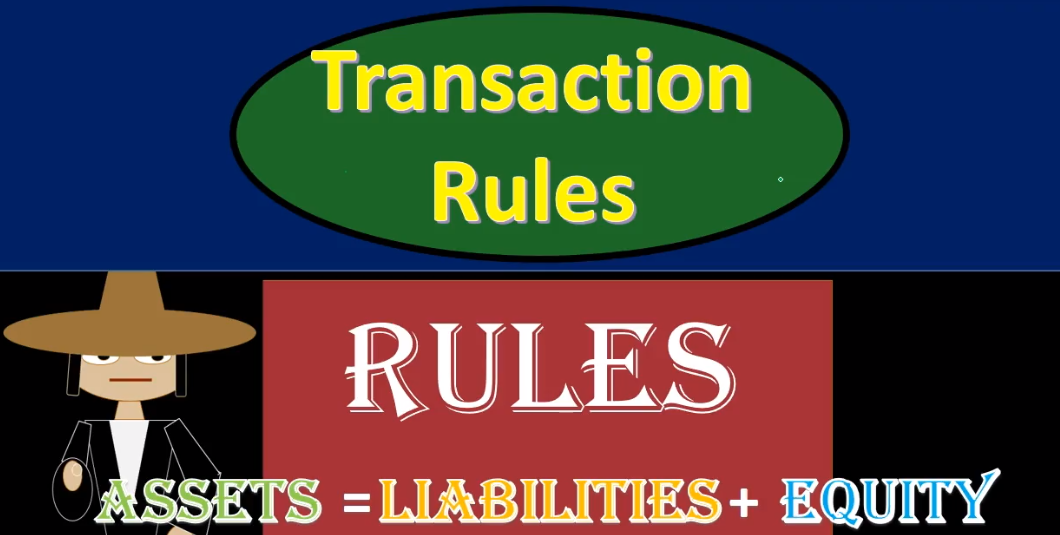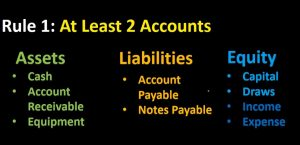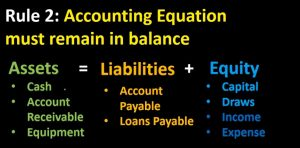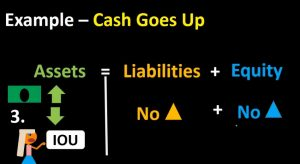## Financial Transaction Rules 155

Hello in this presentation we will be discussing the transaction rules financial transaction rules as they relate to recording financial transactions with regard to the accounting equation. At the end of this, we will be able to list transaction rules explained our reasons for the transaction rules and apply transaction rules to recording financial transactions. First rule, at least two accounts will be affected. It’s going to be whenever we record any transaction and whether we’re talking about a transaction for recording payroll record an accounts receivable, recording accounts payable, all those normal things that the accounting department does on a day to day basis.

00:43

Those are going to be the normal types of transactions that will happen when considering transactions. We often think of transactions in the accounting department as related to documentation such as invoices and bills, and whatnot. And those invoices and bills. When input into the system would then record a transaction that would then be used to generate the financial statements, we here are going to be recording those transactions, those transactions that a system a computerized system would generate in relation to certain type of documentation. Oftentimes, every financial transaction can be represented in terms of the accounting equation, and we’re going to list the rules for understanding what those transactions would be when listing them in terms of the accounting equation. And of course, that first rule is at least two accounts are affected.01:36

Now remember, when we’re looking at the accounting equation, we are looking at assets, liabilities and equity assets equals liabilities and equity. However, these are account types and they are not the actual accounts. When considering assets accounts. We have accounts such as cash, accounts receivable equipment, these are the actual accounts which are under the account type of assets for liabilities, we’re going to have typically accounts payable notes payable the primary accounts we will be starting with when we first start recording transactions. And under equity we have the capital and the draws in the entire income statement, including income or revenue and expenses. Equity remember could be represented as owner’s equity for a sole proprietor partnerships equity for a partnership or a stockholders equity for a corporation.

02:29

However, the total equity section is the equity section, and it represents the book value or net value of the company. Also remember that the capital account with regard to a corporation, we’re going to have different types of accounts within a corporation which would be retained earnings and common stock. And the draws would then be something similar to the dividends and remember that the entire income statement is going to be part of the equity section as we go through these transaction rules. Now, the first rule is that at least two accounts are affected meaning we cannot just have one account affected within any of these categories. Because then the accounting equation would be out of balance, we need at least two there may be more than two, but there will never be less than two.03:16

So whenever recording any transaction, whether with the accounting equation as we will do here, or with debits and credits, remember that there will never be one account only, there will often majority of the time being two accounts. And it’s possible to have more than two accounts as long as we remain in balance. Rule number two, the accounting equation must remain in balance. So the accounting equation is assets equal liabilities plus equity, and we need to record the journal entry in such a way that the accounting equation will remain in balance. That means that we have to have at least two accounts affected in order for that to happen if something happens to one account something Then must happen to another account. For example, if assets go up, then liabilities could go up, equity could go up, or assets could also go down.

04:10

And that would mean that two accounts would have to be affected on one side of the equal sign or one account going up on this side and one account going up on this side, whether that account be liability or equity related. We take a look at some examples. We’re going to go through the examples related to cash and look at the scenarios of what if cash went up? What are the other things that could happen? Well, liabilities could go up as we said, equity couldn’t go up or assets could go up. Let’s take a look at a scenario of each of those types of transactions. We’re going to imagine here, one scenario in that cash is increasing. So there’s an increase in cash, meaning, of course, that assets are increasing cash being an asset, and we’re going to imagine that the second piece of this transaction is that the liabilities are going up due to age. bank loan.05:00

So that’s one type of transaction that could happen, cash would increase and the liability would increase equity remaining the same. And we remain balanced in this case acids going up the amount owed to the bank for money received. And then do of course in the future would be the example of this transaction. Another example of a transaction we’re going to start off with once again cash increasing that same starting point, say what else could happen in order for cash to increase? What if it was the equity side, we could say that equity is increasing. And that could be because income goes up. That’s probably the most common format of equity increasing hopefully as we are receiving income, and therefore assets would go up and equity would go up. In this example. Next example last example.

05:54

We see our same starting point where cash is going up cash being an asset, what else could happen? And in order for our accounting equation to remain in balance, the assets side could also go down with another asset. This is often the most confusing part of the journal, it’s often the most confusing example, when considering assets going up, because in this case, both sides both accounts are on one side of the equal sign, this account being cash, this account being the IOU account, that account called accounts receivable. So we have cash going up, and we’re reducing the account representing what people owe to us. We call that accounts receivable, we’re getting one asset, we’re losing another asset, we’re getting kind of a better asset. In this case, we’re getting cash and losing an IOU to us.06:48

This is one reason the fact that we have two accounts on the same side of the accounting equation that we will move to debits and credits at a later time, because it’s a bit deceiving to record transactions in this format because there’s no net effect on any of the accounts in the accounting equation. Although a transaction took place meaning the net effect on assets is an increase and a decrease, no net effect, no net effect on liabilities, no net effect on equity, although a transaction took place. When we consider this later in terms of debits and credits, we will see that there’ll be a debit and credit for each transaction. And that’s one reason why the debits and credits is going to be a more useful tool when constructing, recording and posting transactions. Objectives. We are now able to list the transaction rules explain reasons for the transaction rules and apply transaction rules to recording transactions.